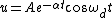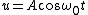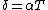# damping

(redirected from Over-damping)
Also found in: Dictionary, Medical.

## Damping

A term broadly used to denote either the dissipation of energy in, and the consequent decay of, oscillations of all types or the extent of the dissipation and decay. The energy losses arise from frictional (or analogous) forces which are unavoidable in any system or from the radiation of energy to space or to other systems. For sufficiently small oscillations, the analogous forces are proportional to the velocity of the vibrating member and oppositely directed thereto; the ratio of force to velocity is -R, the mechanical resistance. For the role of damping in the case of forced oscillations, where it is decisive for the frequency response, See Forced oscillation, Resonance (acoustics and mechanics), Harmonic motion, Oscillation, Vibration

An undamped system of mass m and stiffness s oscillates at an angular frequency &ogr;0 = (s/m)1/2. The effect of a mechanical resistance R is twofold: It produces a change in the frequency of oscillation, and it causes the oscillations to decay with time. If u is one of the oscillating quantities (displacement, velocity, acceleration) of amplitude A, then Eq. (1) holds in the damped case, whereas in the undamped case Eq. (2)

(1)(2)holds. The reciprocal time 1/α in Eq. (1) may be called the damping constant.

The damped angular frequency ωd in Eq. (1) is always less than ω0. According to Eq. (1), the amplitude of the oscillation decays exponentially; the time required for the amplitude to decrease to the fraction 1/e of its initial value is equal to 1/α.

A common measure of the damping is the logarithmic decrement δ, defined as the natural logarithm of the ratio of two successive maxima of the decaying sinusoid. If T is the period of the oscillation, then Eq. (3) holds. Then 1/δ is the number

(3)of cycles required for the amplitude to decrease by the factor 1/e in the same way that 1/α is the time required.

The Q of a system is a measure of damping usually defined from energy considerations. The Q is π times the ratio of peak energy stored to energy dissipated per cycle and is equal to π/δ.

If α in Eq. (1) exceeds ω0, then the system is not oscillatory and is said to be overdamped. If the mass is displaced, it returns to its equilibrium position without overshoot, and the return is slower as the ratio α/ω0 increases. If α = ω0 (that is, Q = 1/2), the oscillator is critically damped. In this case, the motion is again nonoscillatory, but the return to equilibrium is faster than for any overdamped case.

McGraw-Hill Concise Encyclopedia of Physics. © 2002 by The McGraw-Hill Companies, Inc.

## damping

[′dam·piŋ]
(engineering)
Reducing or eliminating reverberation in a room by placing sound-absorbing materials on the walls and ceiling. Also known as soundproofing.
(physics)
The dissipation of energy in motion of any type, especially oscillatory motion and the consequent reduction or decay of the motion.
The extent of such dissipation and decay.
McGraw-Hill Dictionary of Scientific & Technical Terms, 6E, Copyright © 2003 by The McGraw-Hill Companies, Inc.

## damping

The dissipation of energy with time, e.g., the dissipation of energy in a mechanical system whose free oscillations decrease with time, resulting in a decrease in its amplitude of vibration.
McGraw-Hill Dictionary of Architecture and Construction. Copyright © 2003 by McGraw-Hill Companies, Inc.

## damping

A technique for stabilizing an electronic or mechanical device by eliminating unwanted or excessive oscillations.
Copyright © 1981-2019 by The Computer Language Company Inc. All Rights reserved. THIS DEFINITION IS FOR PERSONAL USE ONLY. All other reproduction is strictly prohibited without permission from the publisher.
Site: Follow: Share:
Open / Close